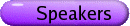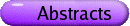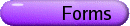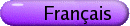Mathematics from Ancient to Modern Times Org: Richard O'Lander and Ronald Sklar (St. John's)[PDF] ROBERT BARONE, Buffalo State College, 1300 Elmwood Avenue, Buffalo, NY  14222 History of Floquet's Characteristic Equation [PDF] A second order ordinary differential equation with real periodic coefficients mathematically describes synthetic or naturally occurring systems that involve periodic structure. The solution to this type of equation was fully investigated by G. Floquet in 1883. Floquet's characteristic equation is an eigenvalue equation that arises in the determination of a solution to the differential equation. Floquet's theorem states that when the roots of the characteristic equation are distinct, the solutions satisfying the differential equation are linearly independent. Floquet's characteristic equation has found wide application in stability theory and wave propagation in periodic structure spanning many fields of science and engineering. We trace the history of Floquet's characteristic equation beginning in the late nineteenth century through the twentieth century and finally into the new millennium. ROBERT DAWSON, Saint Mary's University, Halifax, Nova Scotia "The Hardest Set of Arithmetical Problems in Existence" [PDF] Workman's Tutorial Arithmetic was a high school textbook written around 1900 in Britain that contained what the author referred to as "... I believe, the hardest set of arithmetical problems in existence." This talk will examine some aspects of this textbook, with special consideration of some of the infamous problems, and of what constituted "arithmetic" at that time. JAMES GUYKER, Buffalo State College, 1300 Elmwood Avenue, Buffalo, New York 14222, USA Vector Spaces of Magic Squares [PDF] An interesting example to use to illustrate the notions of linear algebra is the vector space M(n) of real magic squares of order n. In 1910 E. Bergholt obtained a formula for generating magic squares of order four from eight arbitrary real numbers, thereby extending the solution to the ancient problem of constructing one with sixteen consecutive integers. J. Chernick generalized this formula in 1938 in describing a basis for the space M(n) for any n. We show that the orthogonal projection of any n×n matrix onto M(n) has a simple, closed form and its complementary projection may be explicitly described in terms of the projections onto the spaces of affine, coaffine, and doubly affine matrices. SANFORD SEGAL, University of Rochester Helmut Hasse [PDF] After World War I, Helmut Hasse was shunned by many North American mathematicians as a Nazi collaborator. Hasse did support Hitler, and was praised by Nazi functionaries, but the full story of his involvement, which will be sketched, is much more complicated. GEORGE STYAN, McGill University, Montreal Issai Schur (1875-1941) and the Early Development of the Schur Complement: Photographs, Documents and Biographical Remarks [PDF] We comment on the development of the Schur complement from 1812 through 1968 when it was so named and given a notation by Emilie Virginia Haynsworth (1916-1985). The adjectival noun "Schur" was chosen because of the Hilfssatz in the paper by Issai Schur (1875-1941) published in 1917 in the Journal für die reine und angewandte Mathematik (vol. 147, pp. 205-232), in which the Schur determinant formula was introduced. Following some biographical remarks about Issai Schur, we present the Banachiewicz inversion formula for the inverse of a nonsingular partitioned block matrix which was introduced in 1937 by the astronomer Tadeusz Banachiewicz (1882-1954). We note, however, that closely related results were obtained earlier in 1923 by the geodesist Hans Boltz (1883-1947) and in 1933 by Ralf Lohan (b. 1902). We illustrate our findings with several photographs and copies of original documents. [Joint research with Simo Puntanen (University of Tampere) supplementing Chapter 0 in The Schur Complement and Its Applications, Series: Numerical Methods and Algorithms 4 (Fuzhen Zhang, ed.), Springer 2005; see also Issai Schur (1875-1941) and the Early Development of the Schur Complement, with Applications to Statistics and Probability by Simo Puntanen & George P. H. Styan, Report A 346, Dept. of Mathematics, Statistics & Philosophy, University of Tampere, July 2004.] ROBERT THOMAS, University of Manitoba, Winnipeg, MB R3T 2N2 Euclid's Non-Euclidean Geometry [PDF] One sometimes hears it said that Euclid's Elements is a compendium of the mathematical or geometrical results that were known at the time of writing. While Euclid's Conics ought to be a counterexample, it is not extant and so is useless. Another of Euclid's books, his astronomy book the Phaenomena, is not itself a counterexample either because it contains no significant geometrical results. But it makes definite presuppositions of geometrical theorems well outside the material of the Elements. The paper illustrates some of these.